# The MDC Procedure

### Example 18.1 Binary Data Modeling

The MDC procedure supports various multinomial choice models. However, you can also use PROC MDC to estimate binary choice models such as binary logit and probit because these models are special cases of multinomial models.

Spector and Mazzeo (1980) studied the effectiveness of a new teaching method on students’ performance in an economics course. They reported grade point average (gpa), previous knowledge of the material (tuce), a dummy variable for the new teaching method (psi), and the final course grade (grade). A value of 1 is recorded for grade if a student earned the letter grade "A," and 0 otherwise.

The binary logit can be estimated using the conditional logit model. In order to use the MDC procedure, the data are converted as follows so that each possible choice corresponds to one observation:

data smdata;
input gpa tuce psi grade;
datalines;
2.66      20      0        0
2.89      22      0        0
3.28      24      0        0
2.92      12      0        0

... more lines ...


data smdata1;
set smdata;
retain id 0;
id + 1;

/*-- first choice --*/
choice1 = 1;
choice2 = 0;
decision = (grade = 0);
gpa_2 = 0;
tuce_2 = 0;
psi_2 = 0;
output;

/*-- second choice --*/
choice1 = 0;
choice2 = 1;
decision = (grade = 1);
gpa_2 = gpa;
tuce_2 = tuce;
psi_2 = psi;
output;
run;


The first 10 observations are displayed in Output 18.1.1. The variables related to grade=0 are omitted since these are not used for binary choice model estimation.

Output 18.1.1: Converted Binary Data

id decision choice2 gpa_2 tuce_2 psi_2
1 1 0 0.00 0 0
1 0 1 2.66 20 0
2 1 0 0.00 0 0
2 0 1 2.89 22 0
3 1 0 0.00 0 0
3 0 1 3.28 24 0
4 1 0 0.00 0 0
4 0 1 2.92 12 0
5 0 0 0.00 0 0
5 1 1 4.00 21 0

Consider the choice probability of the conditional logit model for binary choice: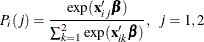The choice probability of the binary logit model is computed based on normalization. The preceding conditional logit model can be converted as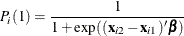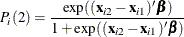Therefore, you can interpret the binary choice data as the difference between the first and second choice characteristics. In the following statements, it is assumed that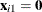. The binary logit model is estimated and displayed in Output 18.1.2.

/*-- Conditional Logit --*/
proc mdc data=smdata1;
model decision = choice2 gpa_2 tuce_2 psi_2 /
type=clogit
nchoice=2
covest=hess;
id id;
run;


Output 18.1.2: Binary Logit Estimates

The MDC Procedure

Conditional Logit Estimates

Parameter Estimates
Parameter DF Estimate Standard
Error
t Value Approx
Pr > |t|
choice2 1 -13.0213 4.9313 -2.64 0.0083
gpa_2 1 2.8261 1.2629 2.24 0.0252
tuce_2 1 0.0952 0.1416 0.67 0.5014
psi_2 1 2.3787 1.0646 2.23 0.0255

Consider the choice probability of the multinomial probit model: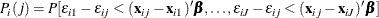The probabilities of choice of the two alternatives can be written as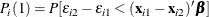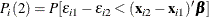where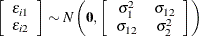. Assume that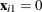and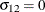. The binary probit model is estimated and displayed in Output 18.1.3. You do not get the same estimates as that of the usual binary probit model. The probabilities of choice in the binary probit model are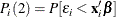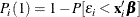where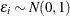. However, the multinomial probit model has the error variance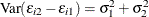if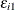and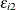are independent (). In the following statements, unit variance restrictions are imposed on choices 1 and 2 (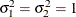). Therefore, the usual binary probit estimates (and standard errors) can be obtained by multiplying the multinomial probit estimates (and standard errors) in Output 18.1.3 by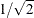.

/*-- Multinomial Probit --*/
proc mdc data=smdata1;
model decision = choice2 gpa_2 tuce_2 psi_2 /
type=mprobit
nchoice=2
covest=hess
unitvariance=(1 2);
id id;
run;


Output 18.1.3: Binary Probit Estimates

The MDC Procedure

Multinomial Probit Estimates

Parameter Estimates
Parameter DF Estimate Standard
Error
t Value Approx
Pr > |t|
choice2 1 -10.5392 3.5956 -2.93 0.0034
gpa_2 1 2.2992 0.9813 2.34 0.0191
tuce_2 1 0.0732 0.1186 0.62 0.5375
psi_2 1 2.0171 0.8415 2.40 0.0165